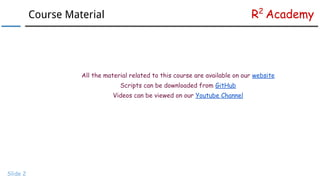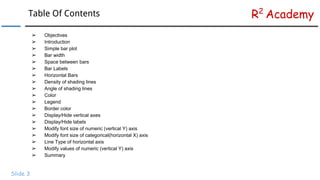Se está descargando tu SlideShare. ×

# R Data Visualization Tutorial: Bar Plots

Anuncio
Anuncio
Anuncio
Anuncio
Anuncio
Anuncio
Anuncio
Anuncio
Anuncio
Anuncio
AnuncioCargando en…3
×

1 de 30 Anuncio

# R Data Visualization Tutorial: Bar Plots

In this tutorial, we learn to create univariate bar plots using the Graphics package in R. We also learn to modify graphical parameters associated with the bar plot.

In this tutorial, we learn to create univariate bar plots using the Graphics package in R. We also learn to modify graphical parameters associated with the bar plot.

Anuncio
Anuncio

### R Data Visualization Tutorial: Bar Plots

1. 1. www.r-squared.in/git-hub R2 Academy Data Visualization With R: Univariate Bar Plots
2. 2. R2 AcademyCourse Material Slide 2 All the material related to this course are available on our website Scripts can be downloaded from GitHub Videos can be viewed on our Youtube Channel
3. 3. R2 AcademyTable Of Contents Slide 3 ➢ Objectives ➢ Introduction ➢ Simple bar plot ➢ Bar width ➢ Space between bars ➢ Bar Labels ➢ Horizontal Bars ➢ Density of shading lines ➢ Angle of shading lines ➢ Color ➢ Legend ➢ Border color ➢ Display/Hide vertical axes ➢ Display/Hide labels ➢ Modify font size of numeric (vertical Y) axis ➢ Modify font size of categorical(horizontal X) axis ➢ Line Type of horizontal axis ➢ Modify values of numeric (vertical Y) axis ➢ Summary
4. 4. R2 AcademyObjectives Slide 4 ➢ Create univariate bar plots ➢ Modify width, space and color of bars ➢ Add legend to the plot ➢ Modify appearance of the axis Note: In this tutorial, we use the Graphics package.
5. 5. R2 AcademyIntroduction Slide 5 A bar chart or bar graph is a chart that presents Grouped data with rectangular bars with lengths proportional to the values that they represent. The bars can be plotted vertically or horizontally. A vertical bar chart is sometimes called a column bar chart. - Wikipedia
6. 6. R2 AcademySimple Bar Plot Slide 6 In the Graphics package, the barplot function is used to create bar plots. You can learn more about this function from the help pages: help(barplot) To create a bar plot, we first tabulate the data using the table function and then use the tabulated data as the input. # basic bar plot data <- table(mtcars\$cyl) barplot(data)
7. 7. R2 AcademyBar Width Slide 7 The width of the bars can be modified using the width option in the barplot function. In the below example, we specify the width of the middle bar to be twice that of the other two bars. # specify width of bars data <- table(mtcars\$cyl) barplot(data, width = c(1, 2, 1))
8. 8. R2 AcademySpace Between Bars Slide 8 The space between the bars can be modified using the space option in the barplot function. In the below example, we specify the space between the bars to be 1.5 # specify space between bars data <- table(mtcars\$cyl) barplot(data, space = 1.5)
9. 9. R2 AcademySpace Between Bars Slide 9
10. 10. R2 AcademyLabels Slide 10 The labels of the bars can be modified using the names.arg option in the barplot function. The names must be specified using a character vector, the length of which should be equal to the number of bars. # specify labels for bars data <- table(mtcars\$cyl) barplot(data, names.arg = c("Four", "Six", "Eight"))
11. 11. R2 AcademyHorizontal Bars Slide 11 Horizontal bar plots can be created using the horiz option in the barplot function. If it is set to TRUE, horizontal bars are drawn else vertical bars are drawn. Note: The default value of this option is FALSE. # horizontal bars data <- table(mtcars\$cyl) barplot(data, horiz = TRUE)
12. 12. R2 AcademyShading Lines (Density) Slide 12 Shading lines can be drawn on the bars using the density option in the barplot function. It takes positive values and the density of the lines increases in proportion to the values specified. Note: The default value of this option is NULL. Lines will not be drawn if negative values are specified. # specify density of shading lines data <- table(mtcars\$cyl) barplot(data, density = 10)
14. 14. R2 AcademyShading Lines (Angle) Slide 14 The angle of the shading lines drawn on the bars can be modified using the angle option in the barplot function. It takes both positive and negative values. # specify angle of shading lines data <- table(mtcars\$cyl) barplot(data, density = 10, angle = 60)
16. 16. R2 AcademyColor Slide 16 The color of the bars can be modified using the col option in the barplot function. The color can be mentioned in RGB or hexadecimal format as well. # specify color of bars data <- table(mtcars\$cyl) barplot(data, col = "blue")
17. 17. R2 AcademyColor Slide 17 Different colors for the bars can be created by specifying a character vector consisting of the names of the colors. If the number of colors mentioned is less than the number of bars, the colors will be repeated. # specify different color for bars data <- table(mtcars\$cyl) barplot(data, col = c("red", "green", "blue"))
18. 18. R2 AcademyLegend Slide 18 A legend can be added using the legend.text option. If it is set to TRUE, a legend is added based on the values associated with the bars. We can also specify a character vector in which case the legend will be created on the basis of the input specified. We will explore legends in more detail in a separate tutorial. # specify legend data <- table(mtcars\$cyl) barplot(data, col = c("red", "green", "blue"), legend.text = TRUE)
19. 19. R2 AcademyBorder Color Slide 19 The border color of the bars can be modified using the border option in the barplot function. The color can be mentioned in RGB or hexadecimal format as well. # specify border color of bars data <- table(mtcars\$cyl) barplot(data, border = "blue")
20. 20. R2 AcademyBorder Color Slide 20 Different colors for the borders can be created by specifying a character vector consisting of the names of the colors. If the number of colors mentioned is less than the number of bars, the colors will be repeated. # specify different border color data <- table(mtcars\$cyl) barplot(data, border = c("red", "green", "blue"))
21. 21. R2 AcademyTitle, Axis Labels & Range Slide 21 We can add a title and modify the axis labels and range using the options we learnt in the earlier tutorials. # specify title, axis labels and range data <- table(mtcars\$cyl) barplot(data, col = c("red", "green", "blue"), main = "Frequency of Cylinders", xlab = "Number of Cylinders", ylab = "Frequency", ylim = c(0, 20))
22. 22. R2 AcademyAxes Slide 22 The vertical axes is not drawn if the axes option is set to FALSE. The default value is TRUE and hence a vertical axes is always drawn unless specified otherwise.
23. 23. R2 AcademyAxis Names Slide 23 The labels of the bars are not added if the axisnames option is set to FALSE. The default value is TRUE and hence the labels are always added unless specified otherwise.
24. 24. R2 AcademyNumeric Axis Font Size Slide 24 The font size of the numeric axis can be modified using the cex.axis option.
25. 25. R2 AcademyLabels Font Size Slide 25 The font size of the labels can be modified using the cex.names option.
26. 26. R2 AcademyAxis Line Type Slide 26 A line type for the horizontal axis can be specified using axis.lty option.
27. 27. R2 AcademyOffset Slide 27 The values of the numeric (vertical Y) axis can be modified using the offset option.
28. 28. R2 Academy Slide 28 ● Bar plots can be created using the barplot() function. ● Tabulate the data using the table() function before plotting. ● Modify the width of the bars using the width option. ● Modify the space between the bars using the space option. ● Modify the labels of the bars using the names.arg option. ● Create horizontal bars using the horiz option. ● Add shading lines using the density option and modify the angle of the lines using the angle option. ● Add color to the bars using the col and border options. ● Add legend to the plot using the legend.text option. ● Display/hide the numeric axis using the axes option. ● Display/hide the labels using the axis.names option. ● Modify font size of the numeric axis using the cex.axis option. ● Modify font size of the labels using the cex.names option. ● Modify line type of the horizontal axis using the axis.lty option. ● Modify values of the numeric axis using the offset option. Summary
29. 29. R2 AcademyNext Steps... Slide 29 In the next module: ✓ Bivariate Bar Plots ✓ Stacked Bar Plots ✓ Grouped Bar Plots
30. 30. R2 Academy Slide 30 Visit Rsquared Academy for tutorials on: → R Programming → Business Analytics → Data Visualization → Web Applications → Package Development → Git & GitHub